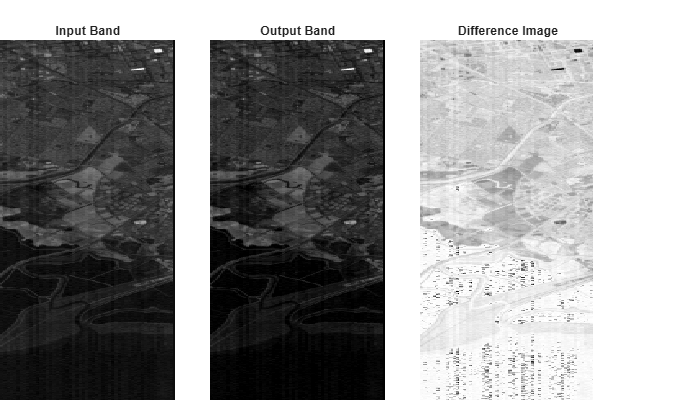# empiricalLine

Empirical line calibration of hyperspectral data

## Syntax

``newhcube = empiricalLine(hcube,imgSpectra,fieldSpectra,fieldWL)``

## Description

example

````newhcube = empiricalLine(hcube,imgSpectra,fieldSpectra,fieldWL)` performs empirical line calibration of the hyperspectral data, `hcube`. The function calculates empirical line factors to force the image spectral data, `imgSpectra`, to match the field reflectance spectra, `fieldSpectra`, with wavelengths `fieldWL`. For more information, see Algorithms. NoteThis function requires the Image Processing Toolbox™ Hyperspectral Imaging Library. You can install the Image Processing Toolbox Hyperspectral Imaging Library from Add-On Explorer. For more information about installing add-ons, see Get and Manage Add-Ons. ```

## Examples

collapse all

Read hyperspectral data into the workspace. This data is from the EO-1 Hyperion sensor, with pixel values in digital numbers.

`hcube = hypercube('EO1H0440342002212110PY_cropped.hdr');`

Remove bad bands from the input data.

`hcube = removeBands(hcube,'BandNumber',find(~hcube.Metadata.BadBands));`

Convert the pixel values from digital numbers to top of atmosphere (TOA) radiance values.

`hcube_toa = dn2radiance(hcube);`

Select a pixel with a low brightness value as the target pixel, and store the pixel value as a cell array. The pixel belongs to the tar region of the input data.

```targetPixel = hcube_toa.DataCube(100,86,:); imgSpec = {permute(targetPixel,[3 1 2])};```

Read the reflectance spectral signature of the tar material from the ECOSTRESS library.

```filename = 'manmade.road.tar.solid.all.0099uuutar.jhu.becknic.spectrum.txt'; info = readEcostressSig(filename);```

Get the field reflectance spectra and the corresponding wavelength information from the metadata of the ECOSTRESS spectral signature. Store these values as cell arrays.

```refSpec = {info.Reflectance}; refWL = {info.Wavelength};```

Perform empirical line calibration of the radiance value hyperspectral data.

`hcube_empirical = empiricalLine(hcube_toa,imgSpec,refSpec,refWL);`

Inspect the results by selecting the same band image from both the uncalibrated digital number hypercube and the calibrated hypercube. For visualization purposes, rescale the values of the two band images to the range [0, 1]. Calculate the absolute value of the difference between the rescaled band images.

```inputBand = rescale(hcube.DataCube(:,:,159)); outputBand = rescale(hcube_empirical.DataCube(:,:,159)); diffBand = abs(inputBand-outputBand);```

Display the original spectral band image, the empirically corrected spectral band image, and the absolute value difference between the band images, to visualize the changes.

```figure('Position',[0 0 700 400]) subplot('Position',[0 0 0.25 0.9]) imagesc(inputBand) title('Input Band') axis off subplot('Position',[0.3 0 0.25 0.9]) imagesc(outputBand) axis off title('Output Band') subplot('Position',[0.6 0 0.25 0.9]) imagesc(diffBand) axis off title('Difference Image') colormap gray ```## Input Arguments

collapse all

Input hyperspectral data, specified as a `hypercube` object. The `DataCube` property of the `hypercube` object stores the hyperspectral data cube.

The input pixel values can be digital numbers, TOA radiance values, or TOA reflectance values. To convert a hypercube containing digital numbers to a hypercube containing TOA radiance or TOA reflectance data, use the `dn2radiance` or `dn2reflectance` function, respectively.

Image spectral data, specified as an N-by-1 cell array. N is the number of pixels or fields used in the empirical line calibration. Each cell contains a C-by-1 numeric vector, where C is the number of hyperspectral bands present in `hcube`.

Field reflectance spectra, specified as an N-by-1 cell array. N is the number of pixels or fields used in the empirical line calibration. Each cell contains a vector of field reflectances. The vectors can vary in size between cells, but the length of the vector in each cell of `fieldSpectra` and `fieldWL` must match.

Wavelength of field reflectance spectra in nanometers, specified as an N-by-1 cell array. N is the number of pixels or fields used in the empirical line calibration. Each cell contains a P-by-1 vector of field wavelengths, of varying lengths. The length of the vector in each cell of `fieldSpectra` and `fieldWL` must match.

## Output Arguments

collapse all

Calibrated hyperspectral data, returned as a `hypercube` object or 3-D numeric array consistent with the input data, `inputData`. The data type of numeric output is `single`. When the input data in `inputData` is of data type `double`, then the corrected data is also of data type `double`. Otherwise, the corrected data is of data type `single`.

## Algorithms

The `empiricalLine` function performs linear regression for each band to equate the digital number (DN), or TOA radiance or TOA reflectance, with the surface reflectance. Solving the linear regression equation provides gain and offset values for each band. This equation shows how the empirical line factors gain and offset values are calculated:

`${\rho }_{surfac{e}_{\lambda }\text{\hspace{0.17em}}=\text{\hspace{0.17em}}m\text{\hspace{0.17em}}{r}_{\lambda }+\text{\hspace{0.17em}}\text{\hspace{0.17em}}offset}$`

The gain (m) and the offset values (offset) are the unknown parameters in the empirical line equation. ρλ is the known surface reflectance value of a reference material in the input hyperspectral data (known as the field reference spectra). rλ is the measured value for the reference material in the input hyperspectral data (known as the image spectral data). The measured value can be a digital number, TOA radiance, or TOA reflectance.

The field reference spectra is an a priori measurement which can also be read from the spectral libraries. The empirical line approach solves the linear equation to find the gain and the offset values. The surface reflectance values for all the other pixels in the input hyperspectral data is calculated using the estimated gain and the offset values.

The `empiricalLine` function automatically resamples the input field spectra to match the selected data wavelengths in `hcube`.

To solve the linear regression equation, at least two field spectrum values must be known for each band. If the `empiricalLine` function is provided with only one field spectrum value for each band, the offset value is set as zero. If there is no field spectrum value available for any of the bands, then this function throws an error.

 Roberts, D. A., Y. Yamaguchi, and R. J. P. Lyon. "Comparison of Various Techniques for Calibration of AIS Data." In Proceedings of the Second Airborne Imaging Spectrometer Data Analysis Workshop, ed. Gregg Vane and Alexander F. H. Goetz, 21–30. Pasadena: Jet Propulsion Laboratory, 1986.

 Kruse, F. A., K. S. Kierein-Young, and J.W. Boardman. "Mineral Mapping at Cuprite, Nevada with a 63-Channel Imaging Spectrometer," Photogrammetric Engineering and Remote Sensing 56, no. 1 (January 1990): 83–92.